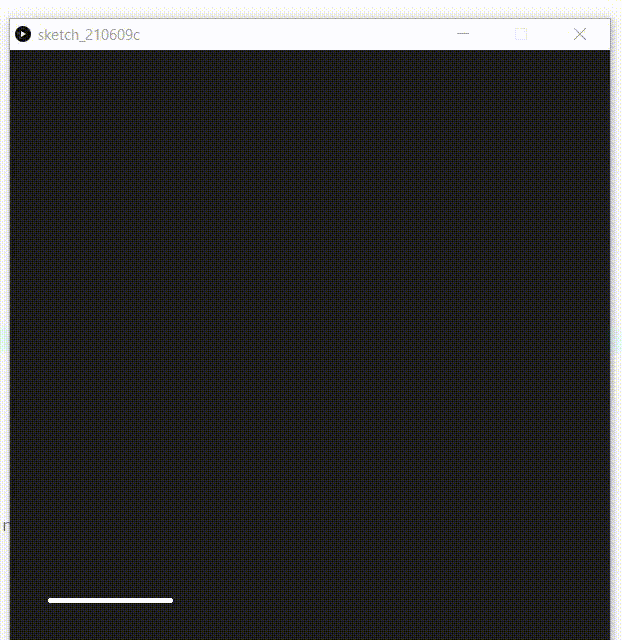# How to draw simple animation using p5.js ?

• Last Updated : 10 Jun, 2022

Animation is a method in which a collection of images is combined in a specific way and processed then they appear as moving images. Building animations make on-screen objects seem to be alive.

In this article, we will learn to make a simple animation of the house in p5.js by using lines, rectangles and ellipses for making the parts of the house.

Approach:

• Make a list to store all the vertices of the house.
• Declare two variable iter and counter.
• Set the function setup() in which the size,colour and background of output window ,initiate the value of iter and counter as 1 and initialise the list of vertices.
• Set the function draw in which add stroke,  stroke weight.
• Make a if condition to check iter within bound if yes increase the counter by 0.05 and take the ceil value of counter as iter , if no exit from the loo.
• Function to add vertices of house giving start and end point of line as addVertices().
• Now make functions to draw the parts of house:
• Make Function to draw vertical and horizontal  lines in house.
• Make Function to draw  square window
• Make Function to draw gate.
• Make Function to draw circular window.
• Make Function to draw chimney.
• After all this step now create a switch case to add all the parts of house step by step.

Below is the implementation of the above approach:

## Javascript

 `// List to store all the vertices` `let vertices = [];`   `// Variable declared` `var` `iter;` `var` `counter;`   `// Function to set up output window` `function` `setup() {`   `    ``// Size of output window` `    ``createCanvas(600, 600);`   `    ``// Fill the color` `    ``fill(31);`   `    ``// Background of output window` `    ``background(31);`   `    ``// Put the value of variables as 1` `    ``iter = 1;` `    ``counter = 1;`   `    ``// Initialize the list of vertices` `    ``addVertices();` `}`   `// Set the draw function` `function` `draw() {`   `    ``stroke(255);` `    ``strokeWeight(4);` `    ``step();`   `    ``// Condition to check within bound` `    ``if` `(iter < 11) {`   `        ``// Increase counter everytime` `        ``counter += 0.05;`   `        ``// Set the iter variable to the` `        ``// floor value of counter` `        ``iter = floor(counter);` `    ``}` `    ``else` `{`   `        ``// If iter increases by 11 then` `        ``// stop the loop` `        ``noLoop();` `    ``}` `}`   `// Function to add vertices of house giving` `// start and end point of line` `function` `addVertices() {` `    ``vertices.push(``new` `p5.Vector(100, 300));` `    ``vertices.push(``new` `p5.Vector(340, 300));` `    ``vertices.push(``new` `p5.Vector(40, 380));` `    ``vertices.push(``new` `p5.Vector(160, 380));` `    ``vertices.push(``new` `p5.Vector(400, 380));` `    ``vertices.push(``new` `p5.Vector(40, 550));` `    ``vertices.push(``new` `p5.Vector(160, 550));` `    ``vertices.push(``new` `p5.Vector(400, 550));` `}`   `// Function to draw lines in house` `function` `drawLine(a, b) {` `    ``line(vertices[a].x, vertices[a].y, ` `        ``vertices[b].x, vertices[b].y);` `}`   `// Function to draw gate` `function` `addGate() {` `    ``rectMode(CENTER);` `    ``rect(100, 500, 70, 100);` `}`   `// Function  to draw window` `function` `addWindow() {` `    ``rect(280, 430, 40, 30);` `}`   `// Function to add  circular window` `function` `addOculus() {` `    ``ellipse(100, 340, 20, 20);` `}`   `// Function to add Chimney` `function` `addChimney() {` `    ``rect(320, 295, 16, 20);` `    ``ellipse(320, 285, 16, 10);` `}`   `// Function to draw parts of ` `// house step by step` `function` `step() {` `    ``switch` `(iter) {` `        ``case` `1:` `            ``drawLine(5, 6);` `            ``break``;` `        ``case` `2:` `            ``drawLine(6, 7);` `            ``break``;` `        ``case` `3:` `            ``drawLine(2, 5);` `            ``drawLine(3, 6);` `            ``break``;` `        ``case` `4:` `            ``drawLine(4, 7);` `            ``break``;` `        ``case` `5:` `            ``drawLine(2, 3);` `            ``drawLine(3, 4);` `            ``break``;` `        ``case` `6:` `            ``drawLine(0, 2);` `            ``drawLine(0, 3);` `            ``drawLine(1, 4);` `            ``break``;` `        ``case` `7:` `            ``drawLine(0, 1);` `            ``break``;` `        ``case` `8:` `            ``addGate();` `            ``break``;` `        ``case` `9:` `            ``addWindow();` `            ``break``;`   `        ``case` `10:` `            ``addOculus();` `            ``break``;` `        ``case` `11:` `            ``addChimney();` `            ``break``;` `    ``}` `}`

Output:My Personal Notes arrow_drop_up
Recommended Articles
Page :